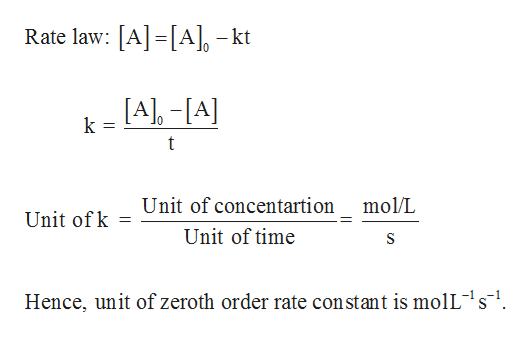# How can I start from the 0th, 1st, and 2nd order rate equations and derive the units for 0th, 1st, and 2nd order rate constants (using moles, liters, and seconds as the basic units)?

Question
2 views

How can I start from the 0th, 1st, and 2nd order rate equations and derive the units for 0th, 1st, and 2nd order rate constants (using moles, liters, and seconds as the basic units)?

check_circle

Step 1

Unit of rate constant (k) can be derived from the rate law. Unit of concentration is “mol/L” whereas unit of time is “t” in the 0th, 1st, and 2nd order rate equations. Units for 0th order rate constant can be derived as follows,help_outlineImage TranscriptioncloseRate law: A[A] -kt [A-[A k t Unit of concentartion mol/L Unit of k Unit of time Hence, unit of zeroth order rate constant is molL1s1. fullscreen
Step 2

Units for 1st order rate constant can...

### Want to see the full answer?

See Solution

#### Want to see this answer and more?

Solutions are written by subject experts who are available 24/7. Questions are typically answered within 1 hour.*

See Solution
*Response times may vary by subject and question.
Tagged in

### Chemistry